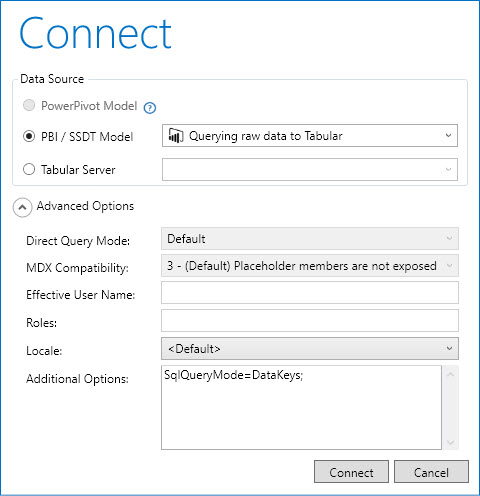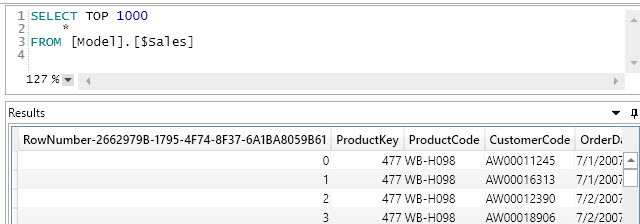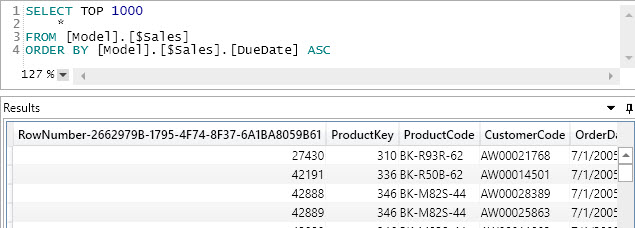When you look at the content of a table in Power BI, SQL Server Data Tools (SSDT) for Visual Studio, or Power Pivot, the queries sent to the Tabular engine use a syntax that guarantees minimal materialization and fast performance. For that purpose, Power BI uses a DAX syntax whereas Analysis Services and Power Pivot use a special SQL syntax.

DISCLAIMER: The content of this article includes information derived from the analysis of queries sent by the existing tools. It is not documented by Microsoft. All these techniques are subject to change in future releases, and might not be supported by Microsoft.

## Querying raw data in DAX

Power BI relies on a DAX query using SELECTCOLUMNS and including all the columns of a table. The undocumented feature is the use of TOPNSKIP to retrieve a number of rows from a table efficiently, skipping a number of rows. Compared to TOPN, the TOPNSKIP function is less flexible, but much faster. This is the syntax of TOPNSKIP:

```TOPNSKIP (
<rows>, -- Number of rows to return
<skip>, -- Number of rows to skip (0 to start from first row)
<table> -- Table expression
[, <orderbyexpression> [,<order>] ]
)
```

For example, this is how Power BI queries the Sales table when you go in Data view. You can try the same query using DAX Studio, and you can monitor what Power BI does by enabling the All Queries trace feature in DAX Studio.

```EVALUATE
SELECTCOLUMNS (
TOPNSKIP ( 1000, 0, 'Sales' ),
"CustomerCode", [CustomerCode],
"DiscountAmount", [DiscountAmount],
"DueDate", [DueDate],
"OrderDate", [OrderDate],
"OrderDateKey", [OrderDateKey],
"OrderQuantity", [OrderQuantity],
"ProductCode", [ProductCode],
"ProductKey", [ProductKey],
"ProductStandardCost", [ProductStandardCost],
"SalesAmount", [SalesAmount],
"ShipDate", [ShipDate],
"TotalProductCost", [TotalProductCost],
"UnitPrice", [UnitPrice]
)
```

The above query returns the first 1,000 rows of the table. If you scroll down the table, you will see this other query appearing in the trace just after showing row number 1,001:

```EVALUATE
SELECTCOLUMNS (
TOPNSKIP ( 1000, 1000, 'Sales' ),
"CustomerCode", [CustomerCode],
"DiscountAmount", [DiscountAmount],
"DueDate", [DueDate],
"OrderDate", [OrderDate],
"OrderDateKey", [OrderDateKey],
"OrderQuantity", [OrderQuantity],
"ProductCode", [ProductCode],
"ProductKey", [ProductKey],
"ProductStandardCost", [ProductStandardCost],
"SalesAmount", [SalesAmount],
"ShipDate", [ShipDate],
"TotalProductCost", [TotalProductCost],
"UnitPrice", [UnitPrice]
)
```

If you enable the “Sort Descending” setting on the OrderDateKey column of the Sales table, this is the query generated to navigate to the middle of the table:

```EVALUATE
SELECTCOLUMNS (
TOPNSKIP ( 1000, 26000, 'Sales', 'Sales'[OrderDateKey], DESC ),
"CustomerCode", [CustomerCode],
"DiscountAmount", [DiscountAmount],
"DueDate", [DueDate],
"OrderDate", [OrderDate],
"OrderDateKey", [OrderDateKey],
"OrderQuantity", [OrderQuantity],
"ProductCode", [ProductCode],
"ProductKey", [ProductKey],
"ProductStandardCost", [ProductStandardCost],
"SalesAmount", [SalesAmount],
"ShipDate", [ShipDate],
"TotalProductCost", [TotalProductCost],
"UnitPrice", [UnitPrice]
)
```

The result of the query includes 1,000 rows after skipping 26,000 rows following the descending sort order defined by the OrderDateKey column. It is not possible to specify more than one column for the sort order. You can apply filters using CALCULATETABLE. This is not supported in Power BI (as of October 2017), but it is available in the syntax. For example, you can get 1,000 rows skipping 2,000 rows from the Sales table ordered by OrderDateKey and filtering only one product using the following query:

```EVALUATE
CALCULATETABLE (
SELECTCOLUMNS (
TOPNSKIP ( 1000, 2000, 'Sales', 'Sales'[OrderDateKey], ASC ),
"CustomerCode", [CustomerCode],
"DiscountAmount", [DiscountAmount],
"DueDate", [DueDate],
"OrderDate", [OrderDate],
"OrderDateKey", [OrderDateKey],
"OrderQuantity", [OrderQuantity],
"ProductCode", [ProductCode],
"ProductKey", [ProductKey],
"ProductStandardCost", [ProductStandardCost],
"SalesAmount", [SalesAmount],
"ShipDate", [ShipDate],
"TotalProductCost", [TotalProductCost],
"UnitPrice", [UnitPrice]
),
'Sales'[ProductKey] = 477
)
```

## Querying raw data in SQL

SQL Server Data Tools (SSDT) for Visual Studio and Power Pivot use a different user interface that generates the query to Tabular through a SQL syntax. This syntax is a subset of the SQL syntax that was already allowed in Analysis Services Multidimensional to query the model, provided you set the SqlQueryMode to DataKeys in the connection string.

NOTE: You should include the string SqlQueryMode=DataKeys; in the connection string. You can do this in the Advanced Options of the Connect dialog box in DAX Studio, as you can see in the following screenshot.For example, the simple syntax to retrieve all the columns of the first 1,000 rows from the Sales table is the following:

```SELECT TOP 1000
[Model].[\$Sales].[CustomerCode],
[Model].[\$Sales].[DiscountAmount],
[Model].[\$Sales].[DueDate],
[Model].[\$Sales].[OrderDate],
[Model].[\$Sales].[OrderDateKey],
[Model].[\$Sales].[OrderQuantity],
[Model].[\$Sales].[ProductCode],
[Model].[\$Sales].[ProductKey],
[Model].[\$Sales].[ProductStandardCost],
[Model].[\$Sales].[SalesAmount],
[Model].[\$Sales].[ShipDate],
[Model].[\$Sales].[TotalProductCost],
[Model].[\$Sales].[UnitPrice]
FROM [Model].[\$Sales]
```

The TOP condition specifies how many rows to include in the result. Any reference follows the [Model] identifier. The table name must be prefixed with \$ and within square brackets if it includes spaces or special characters. The square brackets are also optional for the column name, and are required only for special names. However, it is a good idea to always use square brackets for table and column names.

The complete syntax is the following – curly braces indicate optional elements, whereas angle brackets are placeholders for arguments/names:

```SELECT {SKIP <number_of_rows_to_skip>} {TOP <number_of_rows_to_return>}
{ * | [Model].[\$<table_name>].[<column_name>] { , … } }
FROM [Model].[\$<table_name>]
ORDER BY [Model].[\$<table_name>].[<column_name>] { ASC | DESC }
```

If you use * instead of a list of columns, you get all the columns of the table including the special RowNumber column. The special RowNumber column identifies the physical position of a row in the table (this is not accessible in DAX).As you see, RowNumber is a zero-based index. Do not confuse this number with the row number of the result. In fact, if you sort the data you obtain the RowNumber of the physical position in the raw table – which could be different from the sort order of the physical table in the data source because of the arrangements made by the compression algorithm in VertiPaq.Just to complete the same examples you have already seen in DAX translated to SQL, here is how to skip the first 1,000 rows:

```SELECT SKIP 1000 TOP 1000
[Model].[\$Sales].[CustomerCode],
[Model].[\$Sales].[DiscountAmount],
[Model].[\$Sales].[DueDate],
[Model].[\$Sales].[OrderDate],
[Model].[\$Sales].[OrderDateKey],
[Model].[\$Sales].[OrderQuantity],
[Model].[\$Sales].[ProductCode],
[Model].[\$Sales].[ProductKey],
[Model].[\$Sales].[ProductStandardCost],
[Model].[\$Sales].[SalesAmount],
[Model].[\$Sales].[ShipDate],
[Model].[\$Sales].[TotalProductCost],
[Model].[\$Sales].[UnitPrice]
FROM [Model].[\$Sales]
```

The next query skips 26,000 rows and returns 1,000 rows following the descending sort order defined by the OrderDateKey column.  Also in SQL, it is not possible to specify more than one column for the sort order.

```SELECT SKIP 26000 TOP 1000
[Model].[\$Sales].[CustomerCode],
[Model].[\$Sales].[DiscountAmount],
[Model].[\$Sales].[DueDate],
[Model].[\$Sales].[OrderDate],
[Model].[\$Sales].[OrderDateKey],
[Model].[\$Sales].[OrderQuantity],
[Model].[\$Sales].[ProductCode],
[Model].[\$Sales].[ProductKey],
[Model].[\$Sales].[ProductStandardCost],
[Model].[\$Sales].[SalesAmount],
[Model].[\$Sales].[ShipDate],
[Model].[\$Sales].[TotalProductCost],
[Model].[\$Sales].[UnitPrice]
FROM [Model].[\$Sales]
ORDER BY [Model].[\$Sales].[OrderDateKey] DESC
```

The following query returns 1,000 rows skipping 2,000 rows from the Sales table ordered by OrderDateKey and filtering only one product.

```SELECT SKIP 2000 TOP 1000
[Model].[\$Sales].[CustomerCode],
[Model].[\$Sales].[DiscountAmount],
[Model].[\$Sales].[DueDate],
[Model].[\$Sales].[OrderDate],
[Model].[\$Sales].[OrderDateKey],
[Model].[\$Sales].[OrderQuantity],
[Model].[\$Sales].[ProductCode],
[Model].[\$Sales].[ProductKey],
[Model].[\$Sales].[ProductStandardCost],
[Model].[\$Sales].[SalesAmount],
[Model].[\$Sales].[ShipDate],
[Model].[\$Sales].[TotalProductCost],
[Model].[\$Sales].[UnitPrice]
FROM [Model].[\$Sales]
WHERE [Model].[\$Sales].[ProductKey] = 477
ORDER BY [Model].[\$Sales].[OrderDateKey] ASC
```

## Conclusion

Querying raw data stored in a Tabular model can be useful if you create a tool that needs to quickly browse data, similarly to the Power BI and SSDT user interface when you design a data model. Considering that you can apply filter and sort order on a column, this could be an alternative way to execute a drillthrough operation on a large table in a standard report environment where you control the query generation (such as SQL Server Reporting Services).

SELECTCOLUMNSReturns a table with selected columns from the table and new columns specified by the DAX expressions.

`SELECTCOLUMNS ( <Table> [[, <Name>], <Expression> [[, <Name>], <Expression> [, … ] ] ] )`

TOPNSKIPRetrieves a number of rows from a table efficiently, skipping a number of rows. Compared to TOPN, the TOPNSKIP function is less flexible, but much faster.

`TOPNSKIP ( <Rows>, <Skip>, <Table> [, <OrderBy_Expression> [, [<Order>] [, <OrderBy_Expression> [, [<Order>] [, … ] ] ] ] ] )`

TOPNReturns a given number of top rows according to a specified expression.

`TOPN ( <N_Value>, <Table> [, <OrderBy_Expression> [, [<Order>] [, <OrderBy_Expression> [, [<Order>] [, … ] ] ] ] ] )`

CALCULATETABLEContext transition

Evaluates a table expression in a context modified by filters.

`CALCULATETABLE ( <Table> [, <Filter> [, <Filter> [, … ] ] ] )`